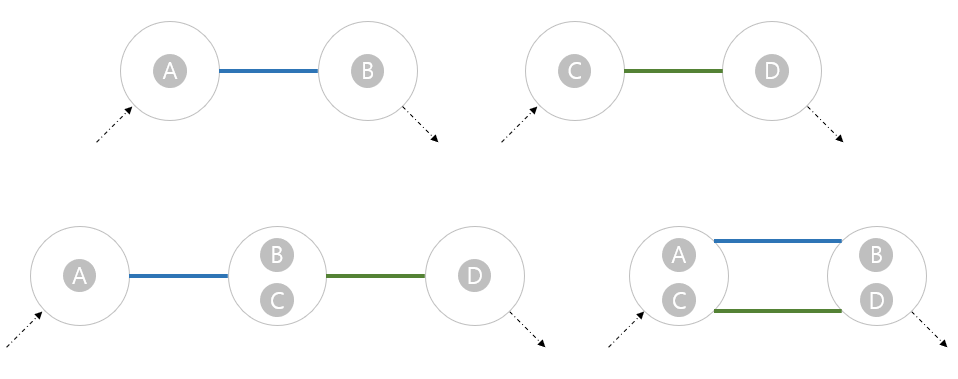시간 제한메모리 제한제출정답맞힌 사람정답 비율
1 초 (추가 시간 없음) 1024 MB72433861.290%

## 문제

Joon is taking General Physics II and he is now studying electronic circuits. An electronic circuit consists of several nodes and undirected wires each connecting two distinct nodes. Moreover, a circuit has two distinctive end nodes; a source node and a sink node, where a voltage is applied (usually it is applied by additional wire with a battery connecting the two nodes, but we will neglect it). Each wire has a resistance, and Joon should know how to calculate the composite resistance of a circuit.

By the way, Joon hates complicated things. So he only cares about circuits that can be made by series and parallel compositions, since they are easy to calculate the composite resistance. He calls them nice circuits; formally, a nice circuit can be defined as follows.

• A circuit with a single wire connecting two end nodes is nice.
• A circuit obtained by merging the sink node of a nice circuit $C_1$ and the source node of a nice circuit $C_2$ into a single node is nice. The source node and the sink node of the obtained circuit are the source node of $C_1$ and the sink node of $C_2$, respectively.
• A circuit obtained by merging the two source nodes of nice circuits $C_1$ and $C_2$ into a single node, and merging the two sink nodes of $C_1$ and $C_2$ into a single node, is nice. The two end nodes of the obtained circuit are the respective merged end nodes.Figure: Illustration of the definition of nice circuit.

He made a circuit with his wires to calculate the composite resistance, but his friend Pringles screwed up his circuit, so now Joon does not know what the end nodes are. To make things worse, he is not even sure whether the circuit is nice or not.

Joon will give you the circuit. He kindly asks you whether the circuit can be nice by appropriately choosing two end nodes. Be careful that there may be multiple wires connecting two nodes.

## 입력

The first line contains two integers, $n$ and $m$ ($2\leq n\leq 100,000$, $1\leq m\leq 300,000$), where $n$ is the number of nodes and $m$ is the number of wires. All nodes are numbered from $1$ to $n$.

In the following $m$ lines, each line contains two integers $u$ and $v$ ($1 \leq u,\ v \leq n$, $u \neq v$), which represents a wire connecting $u$ and $v$. It is guaranteed that every node is attached to at least one wire; otherwise the node does not exist!

## 출력

Print Yes if the given circuit can be nice, or No otherwise.

## 예제 입력 1

4 6
1 2
2 3
2 3
3 4
1 4
1 4


## 예제 출력 1

Yes


## 예제 입력 2

4 6
1 2
1 3
1 4
2 3
2 4
3 4


## 예제 출력 2

No


## 예제 입력 3

9 12
1 9
1 4
5 4
6 5
1 5
8 1
3 6
6 8
3 8
2 9
9 7
7 2


## 예제 출력 3

Yes